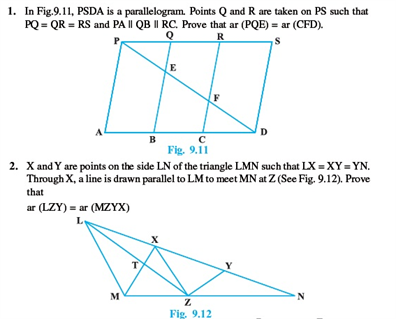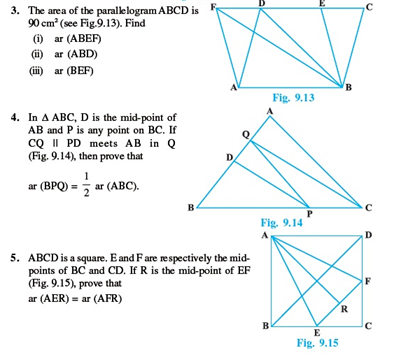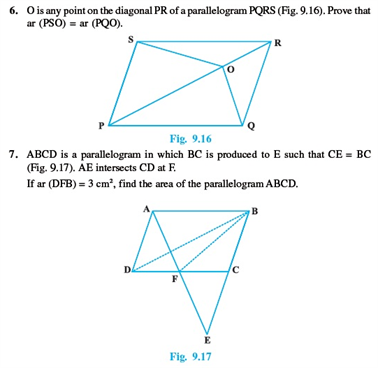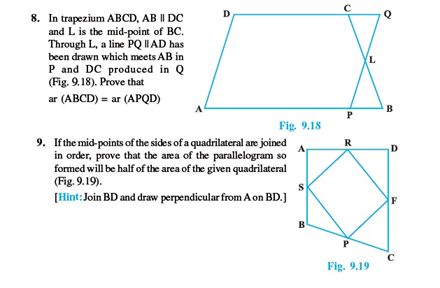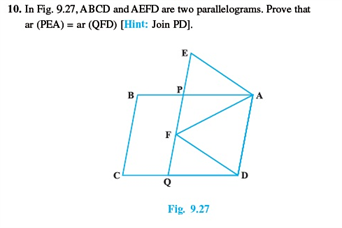# Revision Notes For CBSE Class 9 Math Chapter -9 Areas of Parallelograms and Triangles

A parallelogram is a quadrilateral with two pairs of parallel sides. The opposite sides of a parallelogram are equal in length & opposite angles are equal in measures. The Area of a parallelogram is given by the formulae:

Area = base × height

A triangle is a plane figure with three straight sides and three angles. The sum of angles of a triangle is equal to 180 degrees and the exterior angles of a triangle are always equal to 360 degrees. In a triangle, the sum of the angles is always greater than the length of the third angle. The area of a triangle is given by the formulae:

½ * base * height.

Check here for the free CBSE Class 9 Math revision notes for Chapter 9- Areas of Parallelograms and Triangles